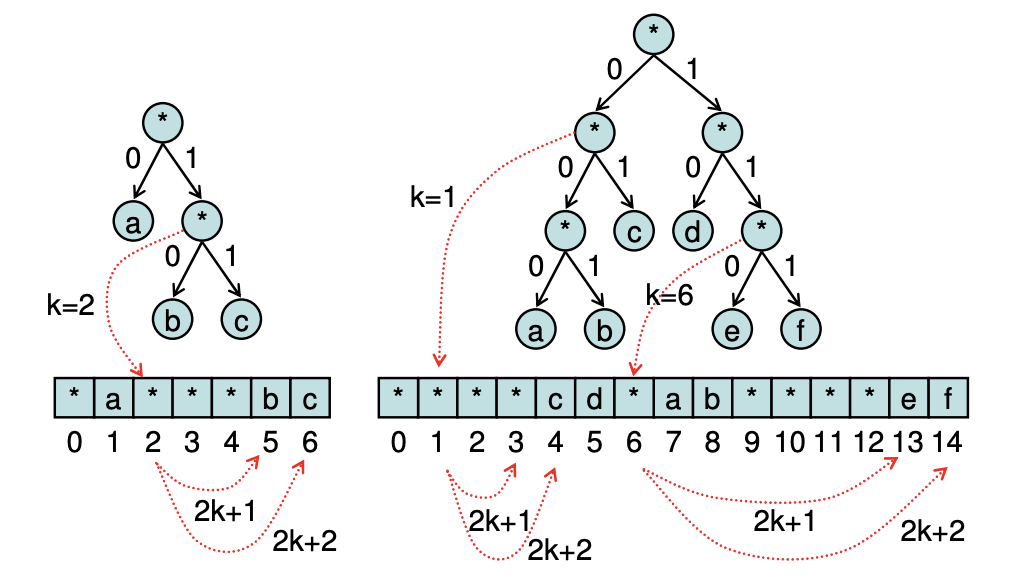시간 제한 메모리 제한 제출 정답 맞은 사람 정답 비율
1 초 128 MB 0 0 0 0.000%

## 문제

Sam has found an ancient communications channel to the outside world. However, the channel is really slow, so Sam and his friends would like to use a compression code to send messages to each other more quickly. After much thought, Sam decides to use a binary prefix code.

A prefix code is a variable-length code system where no valid code in the system is a prefix of any other code. For instance, {a=0, b=10, c=11} is a prefix code, but {a=0, b=10, c=01} is not, since 0 is a prefix of 01.

A binary prefix code (codes consisting of 0’s and 1’s) can be represented as a binary tree, where symbols are leaves and the path from the root to a leaf provides its code. For simplicity we assume the path to the left child contains a 0, and the path to the right child contains a 1. For instance, the binary prefix codes {a=0, b=10, c=11} and {a=000, b=001, c=01, d=10, e=110, f=111} may be represented using the following binary trees.Furthermore, a binary tree may be represented compactly using a string. To see how this works, view a string of length N as a sequence of characters at positions 0 through N-1. Then assume the root node of the tree is at position 0, and the left and right child of a node at position k are at positions 2k+1 and 2k+2, respectively. For instance, the binary trees used earlier may be represented by the strings *a***bc and ****cd*ab****ef, where * is used to represent an interior (non-leaf) or missing node.

Using binary prefix codes, Sam and his friends can compress their messages into binary form for fast transmission. Compressed messages can be decoded easily using the binary tree, starting at the root node and going to either the left or right child, depending on whether the next value is a 0 or 1. If a leaf node representing a character is reached, the character is output and the remainder of the message can be decoded by restarting at the root node.

Your task is to help Sam decode strings encoded as binary numbers back to their original state, using binary prefix codes provided in string format.

## 입력

The first line in the test data file contains the number of test cases (< 100). After that, each line contains one test case. The test case begins with k, the number of strings to be decoded, the string representation of the prefix code, followed by the k strings to be decoded. You may assume all strings to be decoded are composed of characters found in the prefix code.

## 출력

For each test case, you are to output a line containing each decoded string, separated by spaces.

## 예제 입력 1

4
3 *a***bc 0 10 11
2 *a***bc 11010 010100
6 ****cd*ab****ef 000 001 01 10 110 111
5 ****cd*ab****ef 11100001110 000 00100010 01000111110 001110110


## 예제 출력 1

a b c
cab abba
a b c d e f